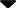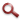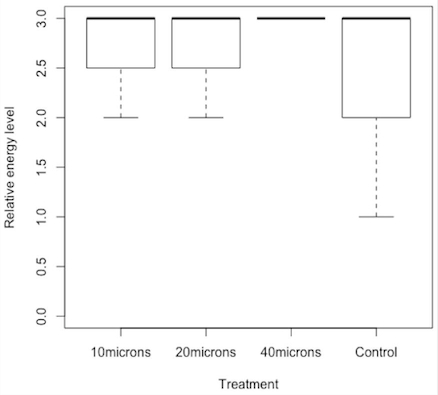Site WebYou are here:

## Student Project FiguresFigure 5 Figure 5: Relative energy levels per treatment. Thick lines of boxplots represent medians. Lower and  upper edges of boxes represent 25% and 75% quartiles, respectively, so that 50% of the energy level values in each treatment are comprised into each box. Extreme lower lines at the ends of dashed vertical lines represent less than 25% of the energy level values for each treatment. Figure 5 Figure 6